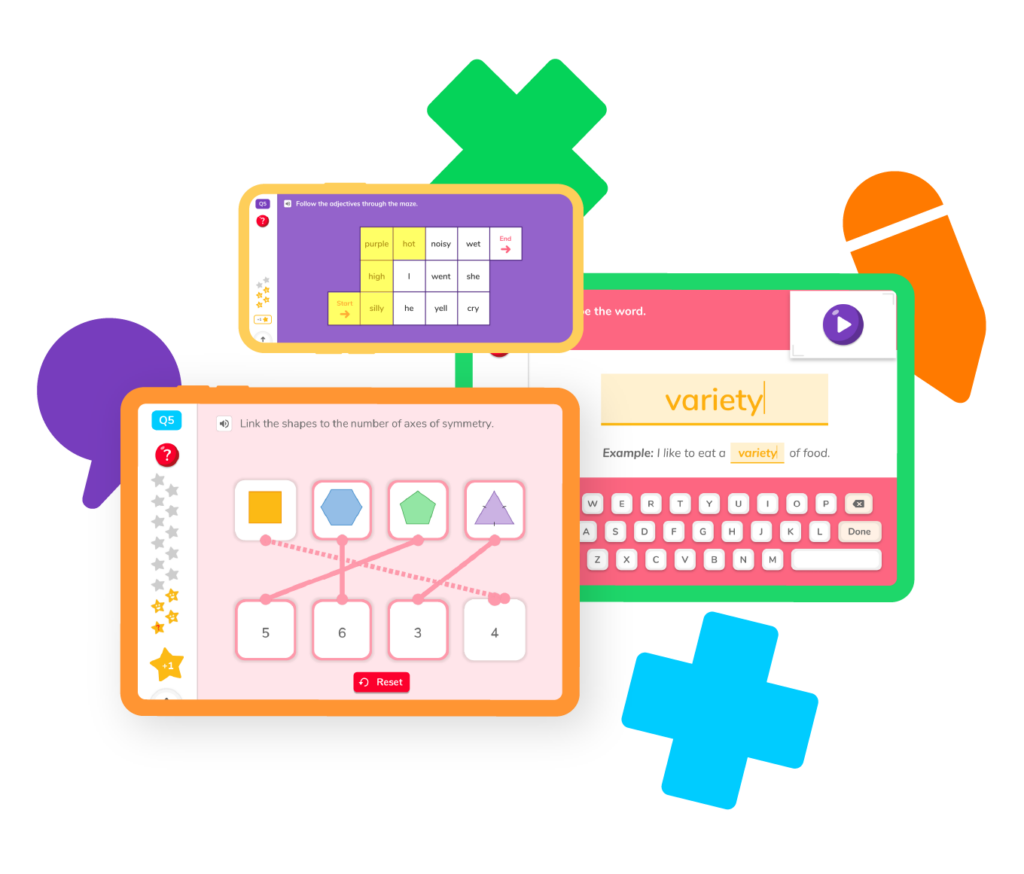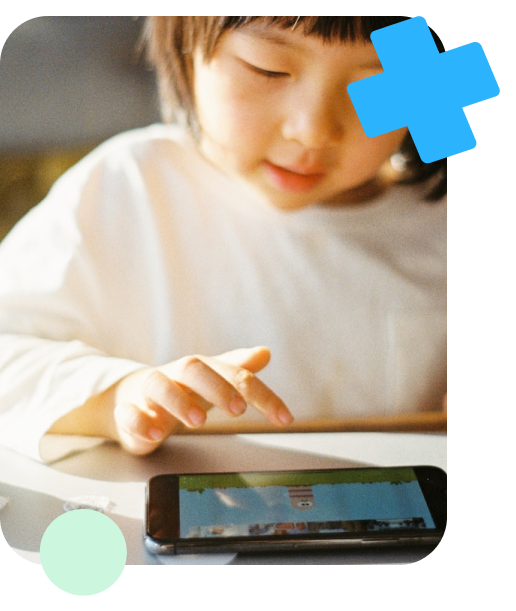Practice important 1st grade math skills like addition, subtraction, measurement, shapes, fractions and more with our games, worksheets, and fun-filled guides. Follow along with classroom activities or scaffold your learning and build a path from least advanced to more advanced topics to become the ultimate math wiz kid!## Select a skillWe’ve gone through the Common Core curriculum and pulled the skills you need to become a pro at 1st grade math! If you’re struggling with addition, enjoy some resources to help catch you up to speed. Or, if you’re looking to get even stronger at your measurement or patterns skills, take a peek and build your confidence!

Learn and practice how to add.

## Subtraction

Learn and practice how to subtract.

## Patterns

Learn how to recognize and create patterns.

## Shapes

Shape up on circles, squares, rectangles and more.

## Time

Practice how to tell time and never be late.

Learn and practice how to add.

## Subtraction

Learn and practice how to subtract.

## Patterns

Learn how to recognize and create patterns.

## Shapes

Shape up on circles, squares, rectangles and more.

## Time

Learn how to identify 2D and 3D shapesStandards vary state by state, but in general, most public schools are expected to follow the Common Core state standards. For 1st graders, the Common Core objectives include:

1. Count to 120. Now that they can recognize numbers and count, 1st graders will extend the counting sequence to 120.
2. Being able to solve addition and subtraction problems within 20. This means students should be able to add and subtract up to sums of 20. They should also be able to use the addition and subtraction symbols and represent addition and subtraction problems using shapes, objects, and drawings.
3. Recognize place value. 1st graders will be able to understand the concept of “tens” and “ones.” So they will know that the number 10 is equal to 10 ones, where the number 20 is equal to 20 ones, etc.
4. Compare the lengths of two objects. They should be able to compare the two lengths of an object by using a third object. So, they should be able to use a ruler to say, “The paper is longer than the pen.”
5. Tell and write time. By the end of 1st grade, your student should be able to look at a clock, tell you the time, and write the time down correctly.
6. Distinguish between what makes a shape and what doesn’t. By the end of 1st grade, students should be able to say that triangles must be closed and have 3 sides, squares must be closed and have 4 sides, but that just because a square and a triangle are the same color, that doesn’t make them the same shape.
7. Compose two- and three-dimensional shapes from composite shapes and vice versa. Essentially, they should know that two-dimensional squares make three-dimensional cubes, and that cubes can be broken down into squares, or that a pyramid can be broken down into triangles with a square on the bottom.Math apps like DoodleMath are a great way to help your student practice math and make it fun at the same time. However, there are several other strategies you can use to help your student grow and learn. Strategies like reading math problems out loud, using props to make concepts like counting and exchanging money seem more concrete, and playing math games can help them grow in their skills and understanding of the subject.

1st graders should understand several types of shapes, including two-dimensional shapes like rectangles, squares, trapezoids, half-circles, and triangles, along with three-dimensional shapes like cubes, cylinders, spheres, cones, and pyramids.

1st grade is typically when students are introduced to basic word problems. It may help them understand word problems if you read them out loud.# Are you a parent, teacher or student?

Are you a parent or teacher?

## Hi there!

Book a chat with our team